# Real Estate Development Model

Deal summary and Cash Flow model

## What is a Real Estate Development Model?

A real estate development model usually consists of two sections: Deal Summary and Cash Flow Model.  Within the Deal Summary, all important assumptions – including the schedule (which lays out the timeline), property stats, development costs, financing assumptions, and sales assumptions – are listed and used to calculate the economics and profitability of the project.

The Cash Flow Model begins with the revenue build up, monthly expenses, financing, and finally levered free cash flows, NPV (net present value) and IRR (internal rate of return) of the project. In the following sections, we will go through the key steps to building a well-organized real estate development model.

### Deal Summary

#### 1. Schedule and Property Stats

The first step in building a real estate development model is to fill in the assumptions for schedule and property stats.  Here is a list of items which should be included: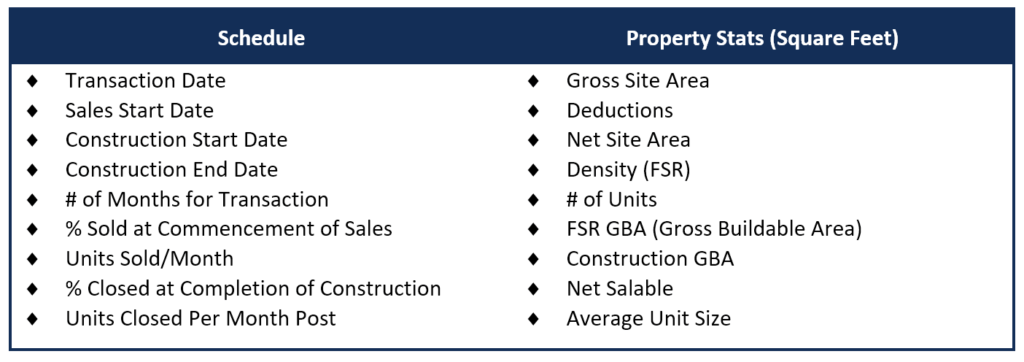#### 2. Development Costs

For the next step in creating real estate development model, we will input the assumptions for development costs in terms of total amount, cost per unit, and cost per square feet.  Development costs might include land cost, building costs, servicing, hard and soft contingency, marketing, etc.  Using the property stats filled in earlier, we can calculate all these numbers and complete the development costs section.  The section should look something like this: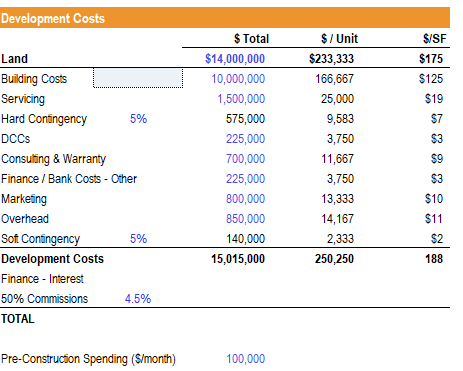Image Source: CFI’s Real Estate Financial Modeling Course.

#### 3. Sales Assumptions

In sales assumptions, we will calculate the total revenue from this project.  Suppose market research is done and based on comparable, we believe that \$500 per square feet is a realistic starting point for the sales price.  We will then use this as the driver for revenue.  After calculating sales (total, \$/unit, \$/SF), sales commissions (e.g. 50%) and warranty, we can figure out the net proceeds from this project.

#### 4. Financing Assumptions

For financing, there are three critical assumptions:  loan to cost percentage, interest rate, and land loan.

Before calculating the total loan amount, we need to figure out the total development cost amount.  Since we have not yet calculated the interest expense, we can link the cell to the cash flow model for now and obtain the value once the cash flow model is filled in.  The commissions are the same as the sales commissions in the sales assumptions section.  The total development costs can be calculated as:

• Total Development Cost = Land Cost + Development Cost + Sum of Interest and Commissions

Now we can fill in the rest of financing assumptions.

• The Max Loan Amount obtained for this project = Total Development Cost x Loan to Cost Percentage
• Equity amount = Total Development Cost – Max Loan Amount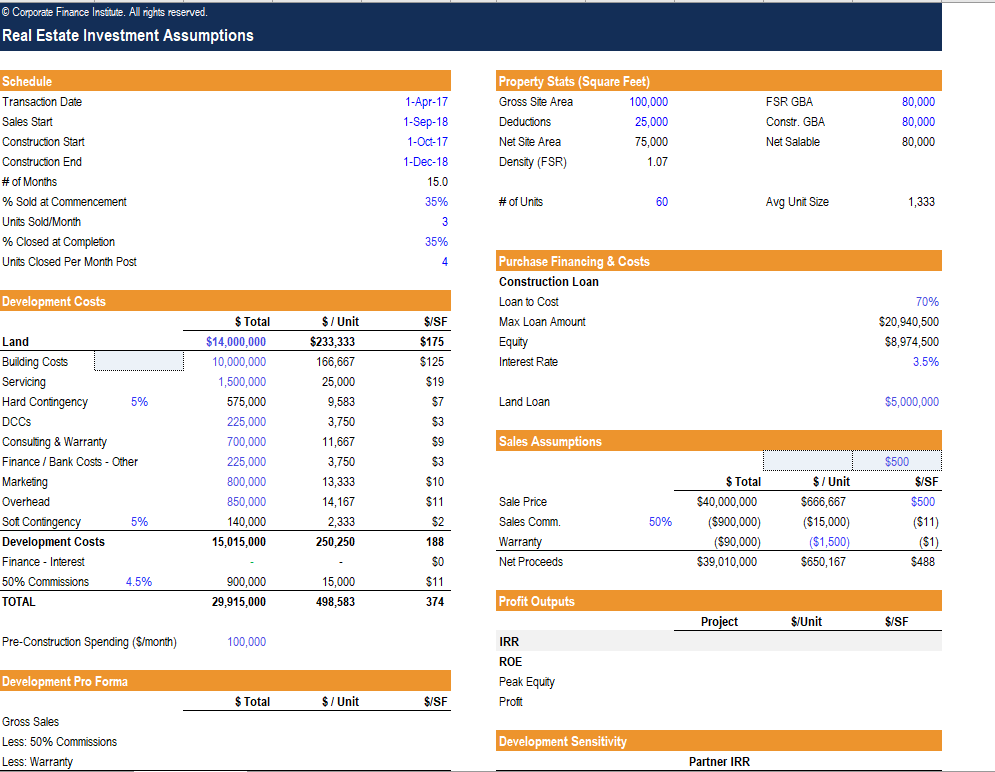Image Source: CFI’s Real Estate Development Model Course.

The figures above will be the assumptions from the Deal Summary section.  Once we complete the Cash Flow Model, we will come back and complete the Development Pro Forma section and sensitivity analysis.

### Cash Flow Model

#### 1. Revenue Build Up

The first step in calculating revenues is to find out the townhome absorption and closings.  Absorption is the number of available homes being sold during a given time period, while closings are the number of homes closed once the construction is complete.

Now we can build up the revenue using the absorption and closings information.

• Townhomes sales = Sales Price/Unit x  Townhome Closings
• First 50% Commissions (charged when homes are sold) = – Townhome absorption x  Sales Price/Unit  x  (Commission% /2)
• Second 50% Commissions (charged when homes are closed) = – Townhome closings x  Sales Price/Unit  x  (Commission% /2)
• Warranty = – Warranty cost/Unit x  Townhome closings
• Total Net Revenue = SUM(Townhome sales + 50% Commissions + 50% Commissions + Warranty)

(*Note that commissions and warranty are in negative amounts.)

#### 2. Expenses

Now, we’ll find out the development expenses, which include land acquisition cost, pre-construction spending, and construction spending.  The numbers can be found in the development costs assumption section from the Deal Summary.

• Land Acquisition Cost = Land cost
• Pre-construction spending = Pre-construction spend (\$/month)
• Construction spending = (Development costs – Pre-construction spending)/No. of months of construction
• Total Development Costs = SUM(Land acquisition cost + Pre-construction spending + Construction spending)

#### 3. Costs to Fund and Proceeds to Repay Capital

The Cost to Fund is the shortfall in the project cash flow that needs to be financed.  When the total net revenue is less than the total development costs, there is a negative cash flow that we need to cover.  When total net revenue becomes greater than the total development costs, then there will be positive proceeds which we can use to pay back capital.  We can use the following formulas to calculate the two numbers:

• Costs to Fund = IF((Total Net Revenue – Total Development Costs) > 0, (Total Net Revenue – Total Development Costs), 0)
• Proceeds to Repay Capital = IF((Total Net Revenue – Total Development Costs) < 0, (Total Net Revenue – Total Development Costs), 0)

#### 4. Financing

Next, we will calculate the loan balances, draws, repayments, and interest accrued.  The table below summarizes the calculations for the first period and the following periods: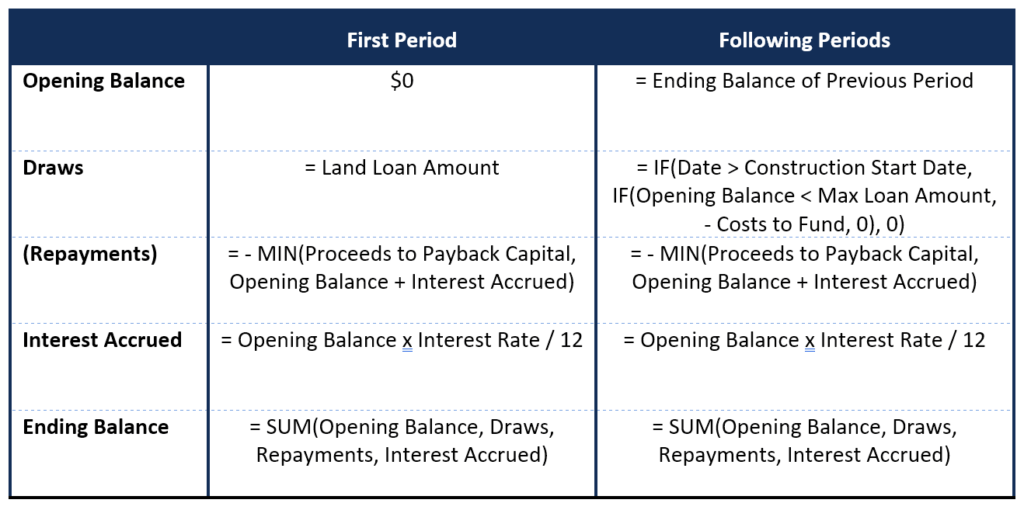We should also perform a quick sanity check to ensure none of the ending balances exceeds the max loan amount.

#### 5. Free Cash Flow and IRR

We can now calculate the levered free cash flows and resulting IRR of this project.

• Levered Free Cash Flow = SUM(Costs to Fund, Proceeds to Payback Capital, Loan Draws, Loan Repayments)

Equity Balance is simply the cumulative FCF:

• The first-period balance = Levered Free Cash Flow
• Following period balances = Previous Balance – Levered Free Cash Flow

Finally using the XIRR formula, we can calculate the Levered IRR for this project:

• Levered IRR =XIRR(All Levered Free Cash Flows, Corresponding Time Period)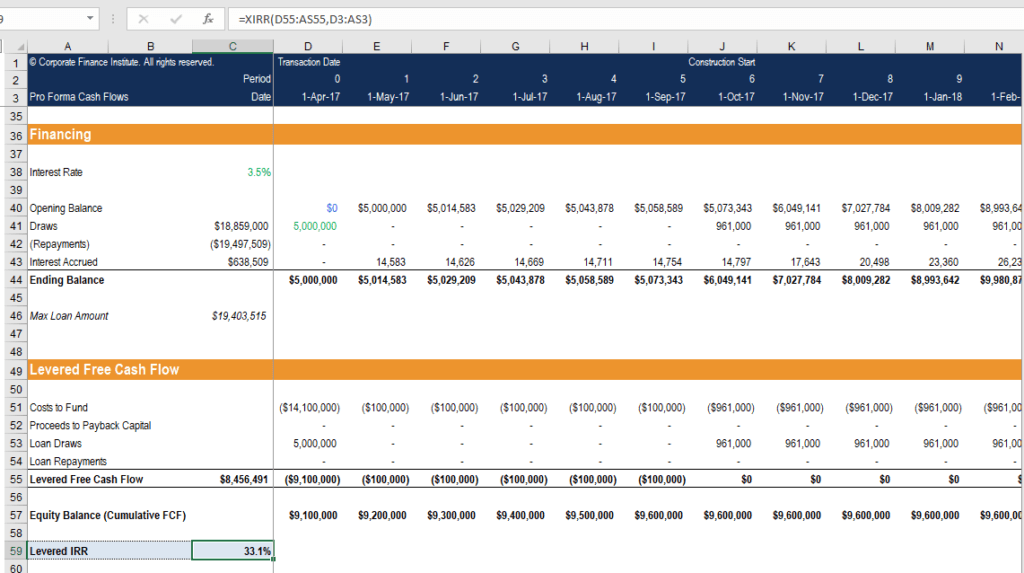Image Source: CFI’s Real Estate Financial Modeling Course.

### More Resources

CFI is the official provider of the Financial Modeling and Valuation Analyst (FMVA)™ certification program, designed to transform anyone into a world-class financial analyst. To keep learning and developing your knowledge of financial analysis, we highly recommend the additional resources below:

• Financial Modeling Best Practices
• Foundations of Real Estate Financial Modeling
• Real Estate Joint Venture
• Types of Financial Models

### Financial Modeling Certification

Become a certified Financial Modeling and Valuation Analyst (FMVA)® by completing CFI’s online financial modeling classes!